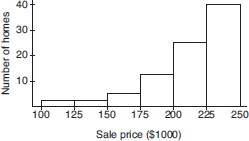# AP Statistics Multiple-Choice Practice Test 21

### Test Information10 questions23 minutes

1. Following is a histogram of 87 home sale prices (in thousands of dollars) in one community:Which of the following could be the median of the sale prices?

2. Which of the following is most useful in establishing cause-and-effect relationships?

3. The average yearly snowfall in a city is 55 inches. What is the standard deviation if 15% of the years have snowfalls above 60 inches? Assume yearly snowfalls are normally distributed.

4. To determine the average cost of running for a congressional seat, a simple random sample of 50 politicians is chosen and the politicians' records examined. The cost figures show a mean of \$125,000 with a standard deviation of \$32,000. Which of the following is the best interpretation of a 90% confidence interval estimate for the average cost of running for office?

5. In one study on the effect that eating meat products has on weight level, an SRS of 500 subjects who admitted to eating meat at least once a day had their weights compared with those of an independent SRS of 500 people who claimed to be vegetarians. In a second study, an SRS of 500 subjects were served at least one meat meal per day for 6 months, while an independent SRS of 500 others were chosen to receive a strictly vegetarian diet for 6 months, with weights compared after 6 months.

6. A plumbing contractor obtains 60% of her boiler circulators from a company whose defect rate is 0.005, and the rest from a company whose defect rate is 0.010. If a circulator is defective, what is the probability that it came from the first company?

7. A kidney dialysis center periodically checks a sample of its equipment and performs a major recalibration if readings are sufficiently off target. Similarly, a fabric factory periodically checks the sizes of towels coming off an assembly line and halts production if measurements are sufficiently off target. In both situations, we have the null hypothesis that the equipment is performing satisfactorily. For each situation, which is the more serious concern, a Type I or Type II error?

8. A coin is weighted so that the probability of heads is 0.75. The coin is tossed 10 times and the number of heads is noted. This procedure is repeated a total of 50 times, and the number of heads is recorded each time. What kind of distribution has been simulated?

9. During the years 1886 through 2000 there were an average of 8.7 tropical cyclones per year, of which an average of 5.1 became hurricanes. Assuming that the probability of any cyclone becoming a hurricane is independent of what happens to any other cyclone, if there are five cyclones in one year, what is the probability that at least three become hurricanes?

10. Given the probabilities P(A) = 0.3 and P(B) = 0.2, what is the probability of the union P(AB) if A and B are mutually exclusive? If A and B are independent? If B is a subset of A?